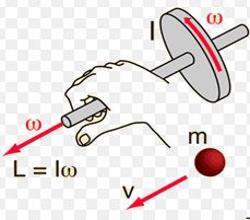Physics

# Explain Conservation of Angular Momentum

The angular momentum of a rotating rigid body is, L = I ω

The torque acting on a rigid body is, τ = dL/dt

When no external torque acts on the system, r = dL/dt = O

L = I ω = constantTotal angular momentum of the body = constant

So, when no external torque acts on the body, the net angular momentum of a rotating rigid body remains constant. This is known as law of conservation of angular momentum.